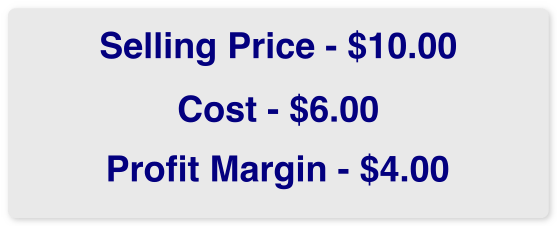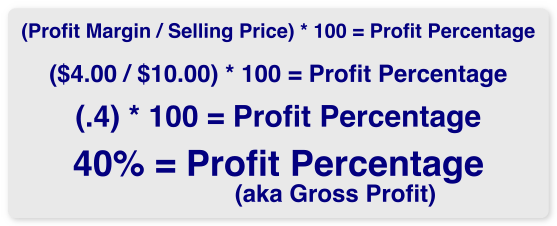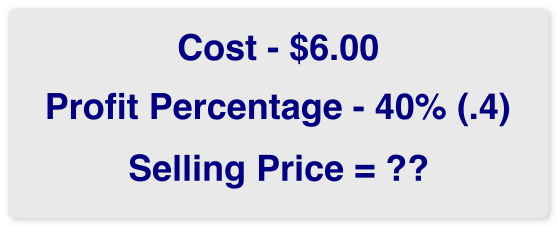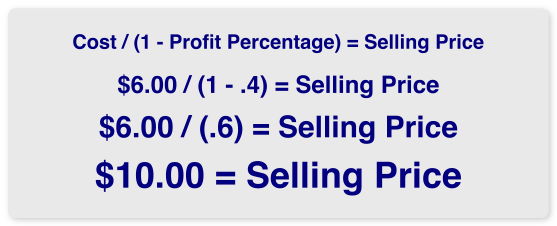Involving yourself in how people price their stuff is like messing with the third rail on the subway.  You do so at your own peril.  So we understand when people tell us that they do their pricing THEIR WAY.  We get that.  We really do.  There are other pricing systems available to Decorators that work differently from the Quote Magician.  Some systems are full management systems with pricing calculators built in.  Some use a Markup or Markon system to compute pricing.  All are valid and have merits.  We decided long ago that the pricing table model is the easiest to use and to understand.  Most importantly, we use the PROFIT MARGIN method to calculate a selling price.  Shown here is an explanation.

As all Promotional Products Distributors know, Margins and Markups/Markons are not the same.  Because the Quote Magician was developed with the Promotional Products Distributor in mind we use the Profit Margin method of pricing.  Simply put, Profit Margin is the amount by which the selling price exceeds costThe math to compute profit margin is simple and straightforward.  To express profit in terms of a percentage the math is also quite simple.So if you know what your cost is, and you know the selling price you can compute the total profit and profit percentage.  But what if you know your cost and you know how much profit (in %) that you want to make – how do you compute selling price?Ahh.  That’s where the Quote Magician excels.  Here is the Super Secret Magic Formula for computing the selling price when you know cost and profit percentage, using the same numbers as our previous example.Ok, so it’s not a Super Secret Magic Formula.  It’s just basic math.  This simple formula is the foundation of the calculations in the Quote Magician.  However, we’ve taken the math a step further.  When you price, for example a printed t-shirt, there are two parts to the “cost”.  The first part is the cost of the blank garment.  The second part is the cost of the decorating.  We give you the ability to apply one profit percentage to the blank garment cost, and apply a different profit percentage to the decorating cost.

What about screen charges, or art charges, or digitizing charges or any other such charges that you have.  Do you apply the same profit percentage to screens?  Do you simply charge \$15.00 or \$20.00 or \$25.00 per screen?  We give you the ability apply a different profit percentage to the setup charges, or no profit at all if you prefer.

And what about oversize garments?  Sometimes XXL, 3XL, etc. can cost way more than the S-XL shirts.  Do you apply the same profit percentage to oversize shirts?  Or do you simply pass along the difference in cost between S-XL and XXL.  For example, if S-XL cost \$1.75 and XXL cost \$4.10, do you simply pass along the difference – \$2.35?  Do you round up a little and charge \$2.50?  Or do you apply your full profit – say 40% and charge \$3.92 more for XXL?

With Quote Magician you have total control over each component of the selling price.